Courses

# Test: Linear Programming Level - 2

## 20 Questions MCQ Test Engineering Mechanics | Test: Linear Programming Level - 2

Description
This mock test of Test: Linear Programming Level - 2 for Mechanical Engineering helps you for every Mechanical Engineering entrance exam. This contains 20 Multiple Choice Questions for Mechanical Engineering Test: Linear Programming Level - 2 (mcq) to study with solutions a complete question bank. The solved questions answers in this Test: Linear Programming Level - 2 quiz give you a good mix of easy questions and tough questions. Mechanical Engineering students definitely take this Test: Linear Programming Level - 2 exercise for a better result in the exam. You can find other Test: Linear Programming Level - 2 extra questions, long questions & short questions for Mechanical Engineering on EduRev as well by searching above.
QUESTION: 1

### Consider the following linear programming problem:Max. Z = 2A + 3B, subject to A + B < 10, 4A + 6B < 30, 2A + B < 17, A, B ≥ 0.What can one say about the solution?

Solution: When one of the constraint is parallel to objective function i.e., if slopes are equal then the LPP may contain multiple optimal solutions.

QUESTION: 2

### The solution for the LPP isMax z = 3x1 + 2x2s/t: 2x1 + 3x2 ≤ 303x1 + 2x2 ≤ 24x1 + x2 ≥ 3x1 , x2 ≥ 0

Solution:

Given,

2x1 + 3x2 ≤ 30 (0,10)(15, 0)

3x1 + 2x2 ≤ 24 (0, 12)(8, 0)

x1 + x2 ≥ 3 (0, 3)(3, 0)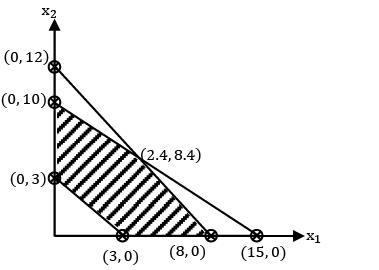z = 3x1 + 2x2

z(3,0) = 9

z(0,3) = 6

z(0,10) = 20

z(2.4,8.4) = 24

z(8,0) = 24

QUESTION: 3

### For a given LPP, Z = 50x1 + 25x2 (max) s/t: 2x1 + 3x2 ≤ 12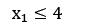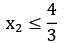x1 , x2 ≥ 0The type of solution obtained is

Solution:

Constraints:

2x1 + 3x2 ≤ 12 (0, 4)(6, 0)

x1 ≤ 4 (x1 = 4)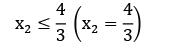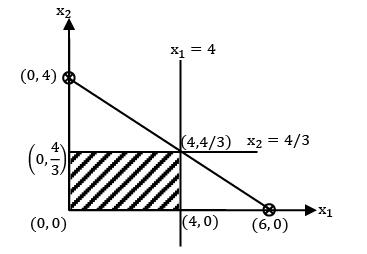z(0,0) = 0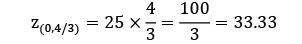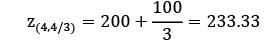z(4,0) = 200

QUESTION: 4

A company produces two products P1 and P2 . The profits per unit of P1 and P2 are Rs.75 and Rs.36 respectively. The market share of the company is 50,000 units of P1 and 80,000 units of P2 .The product P1 require one unit of resource R1 and P2 requires 3 units of resource R1 . The company has a maximum of 60,000 units of R1 . The constraint for resource R1 can be written as

Solution: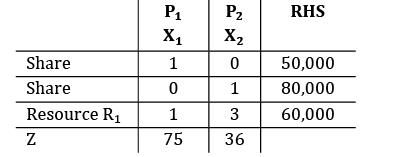X1 + 3X2 ≤ 60, 000

QUESTION: 5

Min z = 4x1 + 6x2

s/t: x1 + x2 ≤ 4

x1 + 2x2 ≥ 6

x1, x2 ≥ 0

The type of solution is

Solution:

Given,

x1 + x2 ≤ 4; (0, 4)(4, 0)

x1 + 2x2 ≥ 6; (0, 3)(6, 0)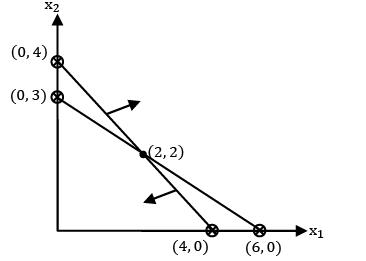only one point:

Z(0,0) = 0

Z(0,3) = 18

Z(2,2) = 20

Z(4,0) = 16

QUESTION: 6

Which of the following type of solution is not possible in simplex method?

Solution:

Simplex method can handle only ≤ type constraints so infeasible solution is not possible.

QUESTION: 7

Which of the following statements are true?

I. Simplex method can handle only ≤ type constraints

II. Simplex method can be applied only when the number of decision variables are ≥ 3

III. Simplex method can be applied to only maximization problems

Solution:
QUESTION: 8

In a transportation problem rows are the supply points and columns are the demand points. If total supply is less than total demand then

Solution:
QUESTION: 9

Which one of the following statements is NOT correct?

Solution:
*Answer can only contain numeric values
QUESTION: 10

If the numbers of sources are 6 and the number of destinations are 7. The total number of non-redundant constraints in linear programming problem formulation is ___________

(A)12

(B)12

Solution:

m+n−1 = 6+7−1 = 12

*Answer can only contain numeric values
QUESTION: 11

Consider the given problem. Find the total cost of transportation using N-W method.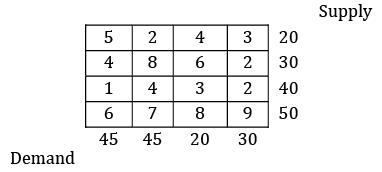Solution: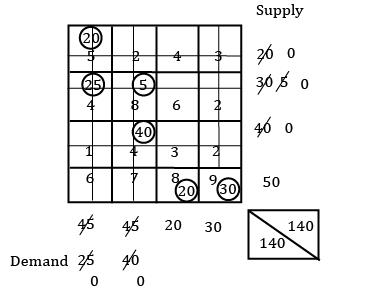TC = 20 × 5 + 25 × 4 + 5 × 8 + 4 × 40 + 8× 20 + 9 × 30

= 830

QUESTION: 12

In order for a transportation matrix which has six rows and four columns, not to be degenerate, how much must be the number of allocated cells in the matrix?

Solution:

m+n−1 = 4+6−1 = 9

QUESTION: 13

Match List-I (O.R. Techniques) with List-II (Application) and select the correct answer using the codes given below the lists: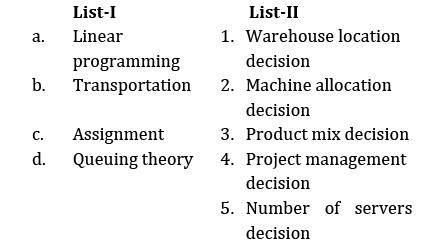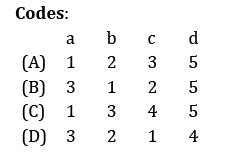Solution:
QUESTION: 14

In a 6 × 6 transportation problem, degeneracy would arise, if the number of filled slots were

Solution:
*Answer can only contain numeric values
QUESTION: 15

Determine the initial basic feasible solution of the following transportation problem by using VAM.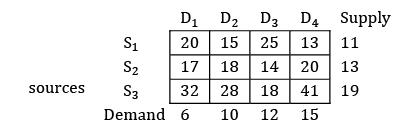Solution: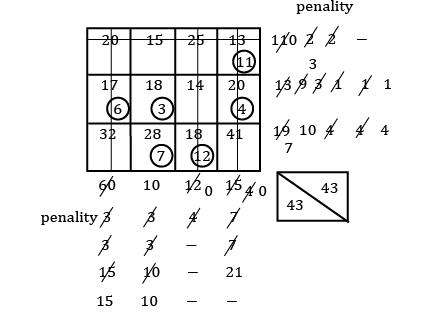TC = 13 × 11 + 6 × 17 + 3 × 18 + 4 × 20 + 7× 28 + 18 × 12 = 791

QUESTION: 16

Consider the following statements: The assignment problem is seen to be the special case of the transportation problem in which

1. m = n

2. All ai = 1

3. xij = 1

(The symbols have usual meaning) Which of the statements given above are correct?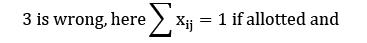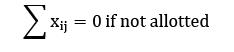Solution:
QUESTION: 17

In an assignment problem having n facilities and n jobs, what is the number of possible ways of making assignments?

Solution:
QUESTION: 18

If there are two constraints in an LPP of which one of the constraints is redundant. The maximum possible number of corner points of the feasible region is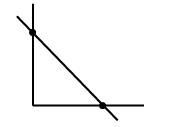As one constraint is redundant it will not touch feasible region.

Solution:
QUESTION: 19

Match the following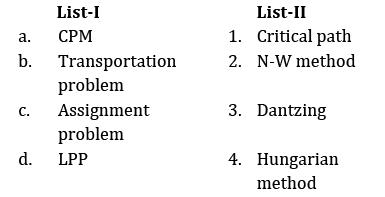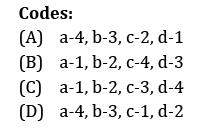Solution:
QUESTION: 20

Consider the following statements regarding linear programming

1. Dual of a dual is the primal.

2. When two minimum ratios of the right hand side to the coefficient in the key column are equal, degeneracy may take place.

Select the correct answer from the codes given below

Codes:

Solution: